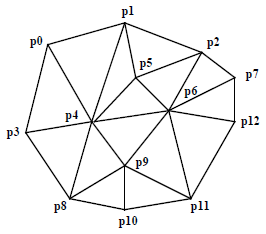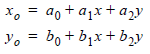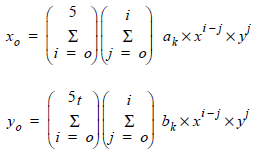# Rubber Sheeting

## Producer Field Guide

HGD_Product
Producer Field Guide
HGD_Portfolio_Suite
Producer

Triangle-Based Finite Element Analysis

The finite element analysis is a powerful tool for solving complicated computation problems which can be approached by small simpler pieces. It has been widely used as a local interpolation technique in geographic applications. For image rectification, the known control points can be triangulated into many triangles. Each triangle has three control points as its vertices. Then, the polynomial transformation can be used to establish mathematical relationships between source and destination systems for each triangle. Because the transformation exactly passes through each control point and is not in a uniform manner, finite element analysis is also called rubber sheeting. It can also be called as the triangle-based rectification because the transformation and resampling for image rectification are performed on a triangle-by-triangle basis.

This triangle-based technique should be used when other rectification methods such as polynomial transformation and photogrammetric modeling cannot produce acceptable results.

Triangulation

To perform the triangle-based rectification, it is necessary to triangulate the control points into a mesh of triangles. Watson (Watson, 1992) summarily listed four kinds of triangulation, including the arbitrary, optimal, Greedy and Delaunay triangulation. Of the four kinds, the Delaunay triangulation is most widely used and is adopted because of the smaller angle variations of the resulting triangles.

The Delaunay triangulation can be constructed by the empty circumcircle criterion. The circumcircle formed from three points of any triangle does not have any other point inside. The triangles defined this way are the most equiangular possible.

The figure below shows an example of the triangle network formed by 13 control points.

Triangle NetworkTriangle-based rectification

Once the triangle mesh has been generated and the spatial order of the control points is available, the geometric rectification can be done on a triangle-by-triangle basis. This triangle-based method is appealing because it breaks the entire region into smaller subsets. If the geometric problem of the entire region is very complicated, the geometry of each subset can be much simpler and modeled through simple transformation.

For each triangle, the polynomials can be used as the general transformation form between source and destination systems.

Linear transformation

The easiest and fastest is the linear transformation with the first order polynomials:There is no need for extra information because there are three known conditions in each triangle and three unknown coefficients for each polynomial.

Nonlinear transformation

Even though the linear transformation is easy and fast, it has one disadvantage. The transitions between triangles are not always smooth. This phenomenon is obvious when shaded relief or contour lines are derived from the DEM which is generated by the linear rubber sheeting. It is caused by incorporating the slope change of the control data at the triangle edges and vertices. In order to distribute the slope change smoothly across triangles, the nonlinear transformation with polynomial order larger than one is used by considering the gradient information.

The fifth order or quintic polynomial transformation is chosen here as the nonlinear rubber sheeting technique in this dissertation. It is a smooth function. The transformation function and its first order partial derivative are continuous. It is not difficult to construct (Akima, 1978). The formula is as follows:It has 21 coefficients for each polynomial to be determined. For solving these unknowns, 21 conditions should be available. For each vertex of the triangle, one point value is given, and two first order and three second order partial derivatives can be easily derived by establishing a second order polynomial using vertices in the neighborhood of the vertex. Then the total 18 conditions are ready to be used. Three more conditions can be obtained by assuming that the normal partial derivative on each edge of the triangle is a cubic polynomial, which means that the sum of the polynomial items beyond the third order in the normal partial derivative has a value zero.

Check Point Analysis

It should be emphasized that the independent check point analysis is critical for determining the accuracy of rubber sheeting modeling. For an exact modeling method like rubber sheeting, the ground control points, which are used in the modeling process, do not have much geometric residuals remaining. To evaluate the geometric transformation between source and destination coordinate systems, the accuracy assessment using independent check points is recommended.